《代数图论》第一章-基本概念## 自同构

$v$在置换$g$下的像记为$v^g.$ 当$g\in \operatorname{Aut}(X)$时, 对子图$Y\subset X,$ 可以保边地定义子图$Y^g\subset X.$ $Y$与$Y^g$自然是同构的.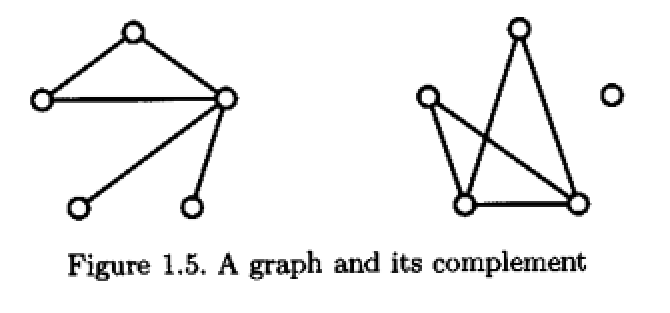## 同态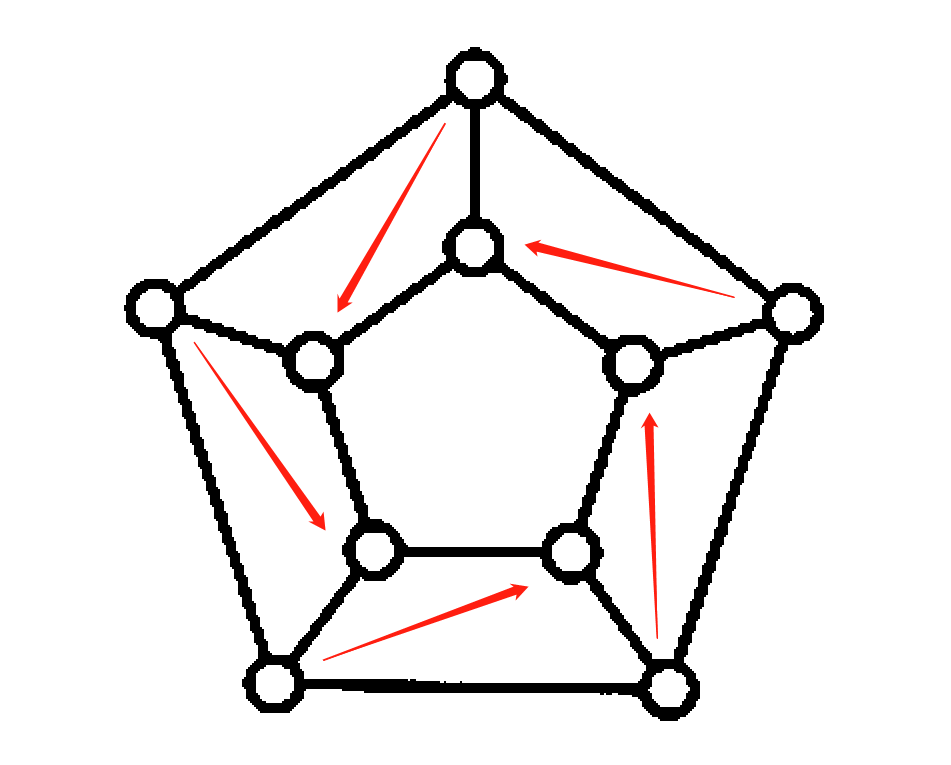## 循环图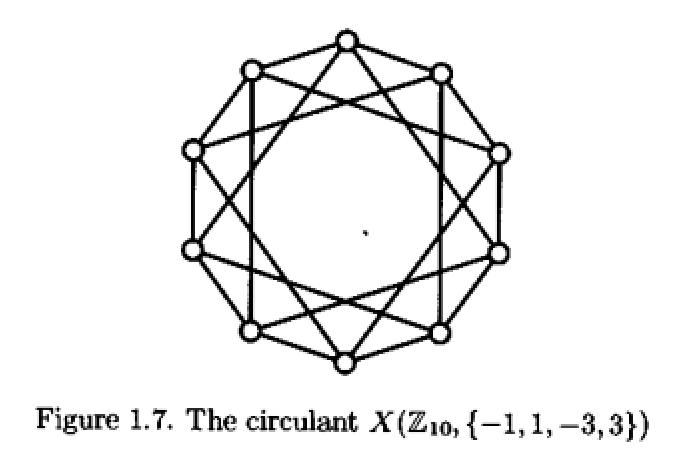## 边图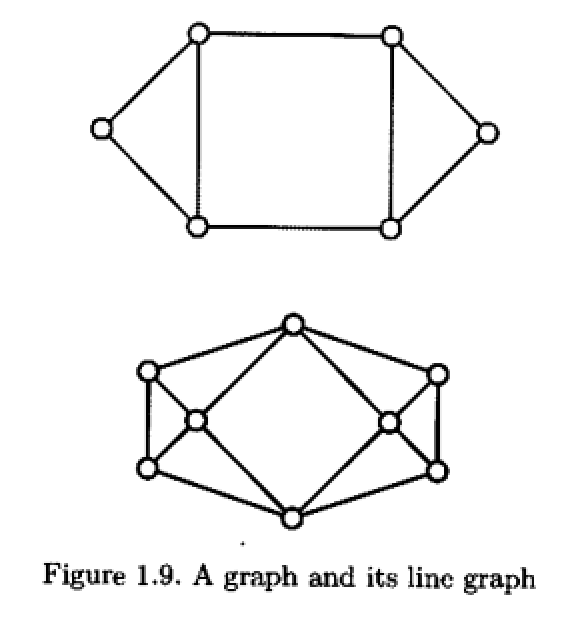$X$中每个度为$k$的顶点都决定了$L(X)$中大小为$k$的团. 若$X$含$n$个顶点, 则$L(X)$中有对应的$n$个团, 使得$L(X)$的每个顶点最多在其中的两个团中, 每条边恰在其中一个团里. 事实上通过构造的方法, 有如下的逆命题:

## 可平面图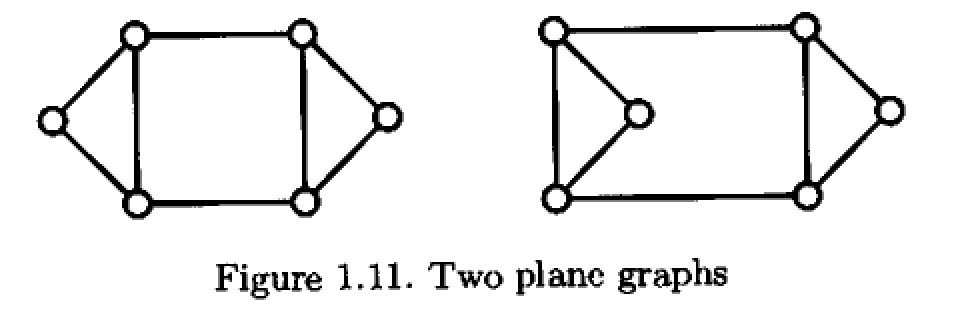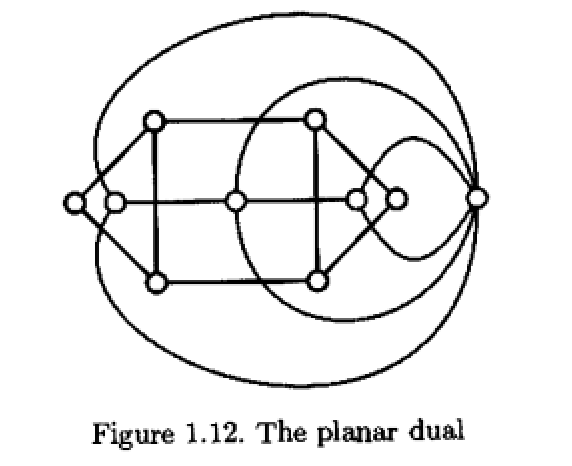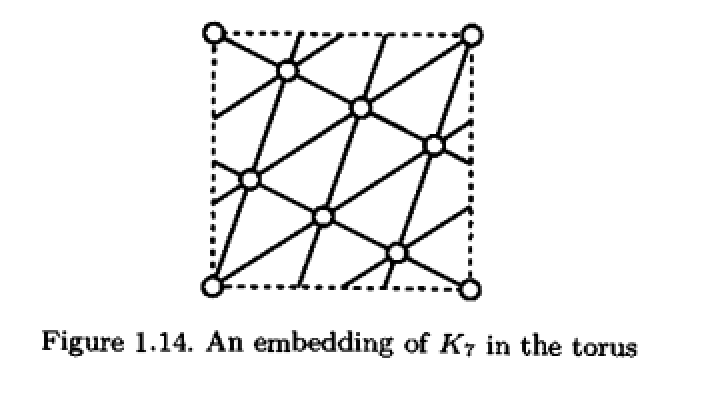• 本文标题：《代数图论》第一章-基本概念
• 本文作者：DreamAR
• 创建时间：2021-09-16 14:51:40
• 本文链接：https://dream0ar.github.io/2021/09/16/《代数图论》第一章-基本概念/
• 版权声明：本博客所有文章除特别声明外，均采用 BY-NC-SA 许可协议。转载请注明出处！
评论